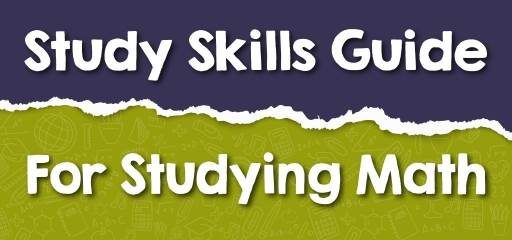# Study Skills Guide For Studying MathMath is different from all other courses we study. It’s not like English or literature, where you can read through or glance through. Mathematics requires active studying. It is objective, and employs the use of formulas and symbols.

You will also agree with me that mathematics speaks a language of its own. There’s no particular way that is deemed perfect for studying Math, but there are some tips and habits you can inculcate to make Math easier for you.

## Math requires active studying

Math is like other courses which someone can read to you, or with just reading, you’re good to go. You have to be alert while studying Math and be away from every form of distraction. It requires actively doing.

You must practice questions and do all your homework. Practicing questions and doing your assignments will allow you to quickly memorize the formulas and procedures used, which will help you become proficient in Math.

Math requires consistent studying, making a study schedule, and allocating adequate time to Math, follow your plan, and you’ll soon see that Math has gotten more straightforward.

## Math accumulates

The process of learning Math is sequential, the topics are not just stacked upon each other, but they follow a progression. One leads to another. The next topic is dependent on the former.

If you want to do well in Math, follow the progress, lagging in the assignment, and studying of, missing classes won’t help, as the lectures keep progressing, you should also progress in your study.

## Study concepts and procedures

Memorizing formulas just won’t cut it. Mathematics has a wide variety of formulas. You need to understand how to use each of them, integrate these formulas into answering questions, and understand the principles involved.

Suppose you cram all the formulas, but you don’t know how to use them. In that case, it’s the same as not knowing the formulas, which is why your focus should be on understanding the concepts of formulas, and equations, and applying mathematical processes.

## Learn the mathematics vocabulary

Mathematics uses a language of its own. Understanding its language will help you understand questions and make answering them easier.

Many commonly used English words have different meanings when integrated into Math questions. For instance, power in mathematics refers to exponent, while it has different meanings in other contexts, such as electrical power in physics.

Also, the difference in mathematics means subtracting two values, while in English, it tells how two things are not alike.

Sometimes this vocabulary has been the source of a barrier to students understanding the subject. To tackle this, you can create a vocabulary log to put down these words and define them. With patience and hard work, you’ll soon discover you like Math.

## Math grows in complexity

As you’re moving from one topic to the other, it starts looking like those questions have gotten more complicated. This results in more study time alluded to Math.

## Take notes

Unless otherwise stated by your lecturer, you should take notes of every theory, example, principle, and explanation given by your lecturer. Also, note every question and formula your lecturer emphasizes

### What people say about "Study Skills Guide For Studying Math - Effortless Math: We Help Students Learn to LOVE Mathematics"?

No one replied yet.

X
30% OFF

Limited time only!

Save Over 30%

SAVE \$5

It was \$16.99 now it is \$11.99